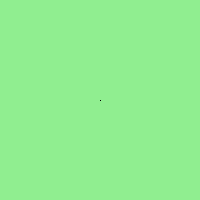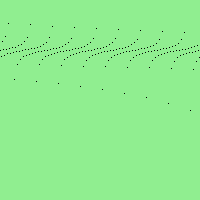Skip to content
Related Articles
Wand point() function in Python
• Last Updated : 01 Jun, 2021

point() is another drawing function and simplest of all. point() function basically draw a point on a particular point on an image. It simply takes two x, y arguments for the point coordinate.

Syntax :

`wand.drawing.point(x, y)`

Parameters:

Example #1:

## Python3

 `# Import different modules of wand``from` `wand.image ``import` `Image``from` `wand.drawing ``import` `Drawing``from` `wand.color ``import` `Color` `# object for Drawing``with Drawing() as draw:``    ``x ``=` `100``    ``y ``=` `100``    ``# draw point at (100, 100) using point() function``    ``draw.point(x, y)` `    ``with Image(width ``=` `200``, height ``=` `200``,``               ``background ``=` `Color(``'lightgreen'``)) as image:` `        ``draw(image)``        ``image.save(filename ``=``"point.png"``)`

Output:Example #2:

## Python3

 `# Import different modules of wand``from` `wand.image ``import` `Image``from` `wand.drawing ``import` `Drawing``from` `wand.color ``import` `Color``import` `math` `with Drawing() as draw:``    ``for` `x ``in` `xrange``(``0``, ``200``):``        ``y ``=` `math.tan(x) ``*` `4``        ``# draw points at different locations using point() function``        ``draw.point(x, y ``+` `50``)``    ``with Image(width ``=` `200``, height ``=` `200``, background ``=` `Color(``'lightgreen'``)) as image:``        ``draw(image)``        ``image.save(filename ``=` `"points.png"``)`

Output:Attention geek! Strengthen your foundations with the Python Programming Foundation Course and learn the basics.

To begin with, your interview preparations Enhance your Data Structures concepts with the Python DS Course. And to begin with your Machine Learning Journey, join the Machine Learning – Basic Level Course

My Personal Notes arrow_drop_up